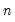vdecomp
Variance decomposition in VARs.
Syntax
var_name.vdecomp(n, options) series_list [@ @ ordering]
List the series names in the VAR whose variance decomposition you would like to compute. You may optionally specify the ordering for the factorization after two “@”-signs.
You must specify the number of periodsover which to compute the variance decompositions.
Options

 g Display combined graphs, with the decompositions for each variable shown in a graph. m Display multiple graphs, with each response-shock pair shown in a separate graph. t (default) Show numerical results in table. imp=arg (default=“chol”) Type of factorization for the decomposition: “chol” (Cholesky with d.f. correction), “mlechol” (Cholesky without d.f. correction), “struct” (structural). The structural factorization is based on the estimated structural VAR. To use this option, you must first estimate the structural decomposition; see Var::svar.The option “imp=mlechol” is provided for backward compatibility with EViews 3.x and earlier. se=mcarlo Monte Carlo standard errors. You must specify the number of replications with the “rep=” option. Currently available only when you have specified the Cholesky factorization (using the “imp=chol” option). rep=integer Number of Monte Carlo replications to be used in computing the standard errors. Must be used with the “se=mcarlo” option. cilevels=arg (default = “0.95”) Confidence interval coverage: space limited list of numbers between 0 and 1. uselines Use lines instead of shading for confidence intervals. matbys=name Save responses by shocks (impulses) in named matrix. The first column is the response of the first variable to the first shock, the second column is the response of the second variable to the first shock, and so on. matbyr=name Save responses by response series in named matrix. The first column is the response of the first variable to the first shock, the second column is the response of the first variable to the second shock, and so on. prompt Force the dialog to appear from within a program. p Print results.
If you use the “matbys=” or “matbyr=” options to store the results in a matrix, two matrices will be returned. The matrix with the specified name contains the variance decompositions, while the matrix with “_FSE” appended to the name contains the forecast standard errors for each response variable. If you have requested Monte Carlo standard errors, there will be a third matrix with “_SE” appended to the name which contains the variance decomposition standard errors.
Examples
var var1.ls 1 4 m1 gdp cpi
var1.vdecomp(10,t) gdp
The first line declares and estimates a VAR with three variables and lags from 1 to 4. The second line tabulates the variance decompositions of GDP up to 10 periods using the ordering as specified in VAR1.
var1.vdecomp(10,t) gdp @ @ cpi gdp m1
performs the same variance decomposition as above using a different ordering.
Cross-references
See “Variance Decomposition” for additional details.